Courses

# Venn Diagrams JEE Notes | EduRev

## JEE : Venn Diagrams JEE Notes | EduRev

The document Venn Diagrams JEE Notes | EduRev is a part of the JEE Course Mathematics (Maths) Class 11.
All you need of JEE at this link: JEE

D. THE PROBABILITY THAT AN EVENT DOES NOT HAPPEN

If, in a possibility space of n equally likely occurrences, the number of times an event  A  occurs is r, there are n - r occasions when A does not happen. 'The event A does not happen’ is denoted by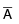(and is read as ‘not A’)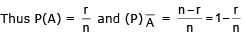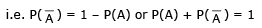This relationship is most useful in the ‘at least one’ type of problem, as is illustrated below.

Example : If four cards are drawn at random from a pack of fifty-two playing cards find the probability that at least one of them is an ace.
If A is a combination of four cards containing at least one ace. (i.e. either one ace, or two aces, or three aces or four aces) thenis a combination of four cards containing no aces.
Now P () =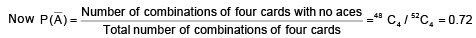Using P (A ) + P () = 1 we have P(A) = 1 - P () = 1 - 0.72 = 0.28

E. VENN DIAGRAMS
A diagram used to illustrate relationships between sets. Commonly, a rectangle represents the universal set and a circle within it represents a given set (all members of the given set are represented by points within the circle). A subset is represented by a circle within a circle and union and intersection are indicated by overlapping circles. Let S is the sample space of an experiment and A, B, C are three events corresponding to it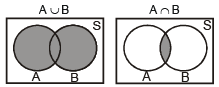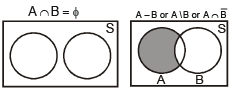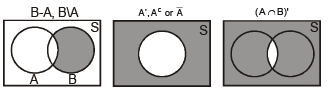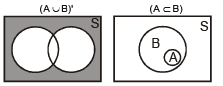Example : Let us now conduct an experiment  of tossing a pair of dice. Two events defined on the experiment are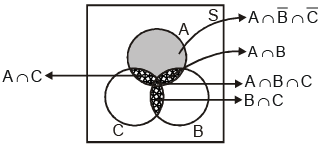A : getting a doublet  {11, 22, 33, 44, 55, 66}
B : getting total score of 10 or more     {64, 46, 55, 56, 65, 66}

A ∪ B = A + B = A or B denotes occurrence of at least A or B.
For 2 events A & B : P (A ∪ B) = P (A) + P (B) - P (A ∩ B)

Note :

 (a) P( A ∪ B)P(A + B)P(A or B)P (occurrence of atleast one A or B) P(A) + P(B) – P( A ∩ B) (This is known as generalized addition theorem)P(A) + P(B ∩) = P(B) + P(A ∩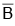)P(A ∩ B ) + P(A ∩ B) + P(B ∩)1 - P (∩)1 - P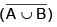(b)  P(A ∪ B) = P(A .) + P(. B) + P(A . B) = 1-P(.) (c) Opposite of “atleast A or B” is neither A nor B i.e.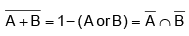Note that P(A + B) + P(∩) = 1.

(d) If A & B are mutually exclusive then P( A ∪ B) = P( A ) + P(B) .
(e) For any two events A & B, P (exactly one of A, B occurs) =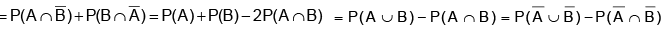(f) De Morgan’s Law : If A & B are two subsets of a universal set U, then
(i)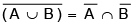(ii)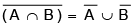(g) A ∪ (B ∩ C) = (A ∪ B) ∩ (A ∪ C) & A ∩ (B ∪ C) = (A ∩ B) ∪ (A ∩ C)

Ex.10 A bag contains 6 white, 5 black and 4 red balls. Find the probability of getting either a white or a black ball in a single draw.
Sol.
Let A = event that we get a white ball, B = event that we get a black ball So, the events are mutually exclusive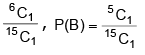So P( A + B) = P( A ) + P(B) =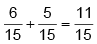Ex.11 Three numbers are chosen at random without replacement from 1, 2, 3, ......, 10. The probability that the minimum of the chosen numbers is 4 or their maximum is 8, is

Sol. The probability of 4 being the minimum number =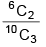(because, after selecting 4 any two can be selected from 5, 6, 7, 8, 9, 10).
The probability of 8 being the maximum number =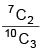The probability of 4 being the minimum number and 8 being the maximum number =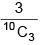∴   the required probability = P( A ∪ B) = P( A ) + P(B) - P( A ∩ B)  =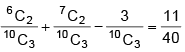Ex.12 A pair of dice is rolled together till a sum of either 5 or 7 is obtained. Find the probability that 5 comes before 7.
Sol.
Let E1 = the event of getting 5 in a roll of two dice = {(1, 4), (2, 3), (3, 2), (4, 1)}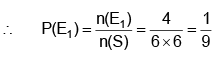Let E2 =  the event of getting either 5 or 7 = {(1,4), (2,3), (3,2),(4,1), (1, 6), (2, 5), (3, 4), (4, 3), (5, 2), (6, 1)}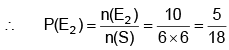∴    the probability of getting neither 5 nor 7 =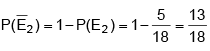The event of getting 5 before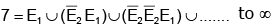∴ the probability of getting 5 before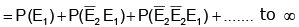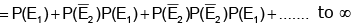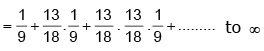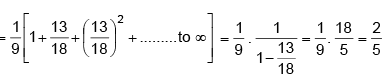Offer running on EduRev: Apply code STAYHOME200 to get INR 200 off on our premium plan EduRev Infinity!

## Mathematics (Maths) Class 11

158 videos|186 docs|161 tests

,

,

,

,

,

,

,

,

,

,

,

,

,

,

,

,

,

,

,

,

,

;